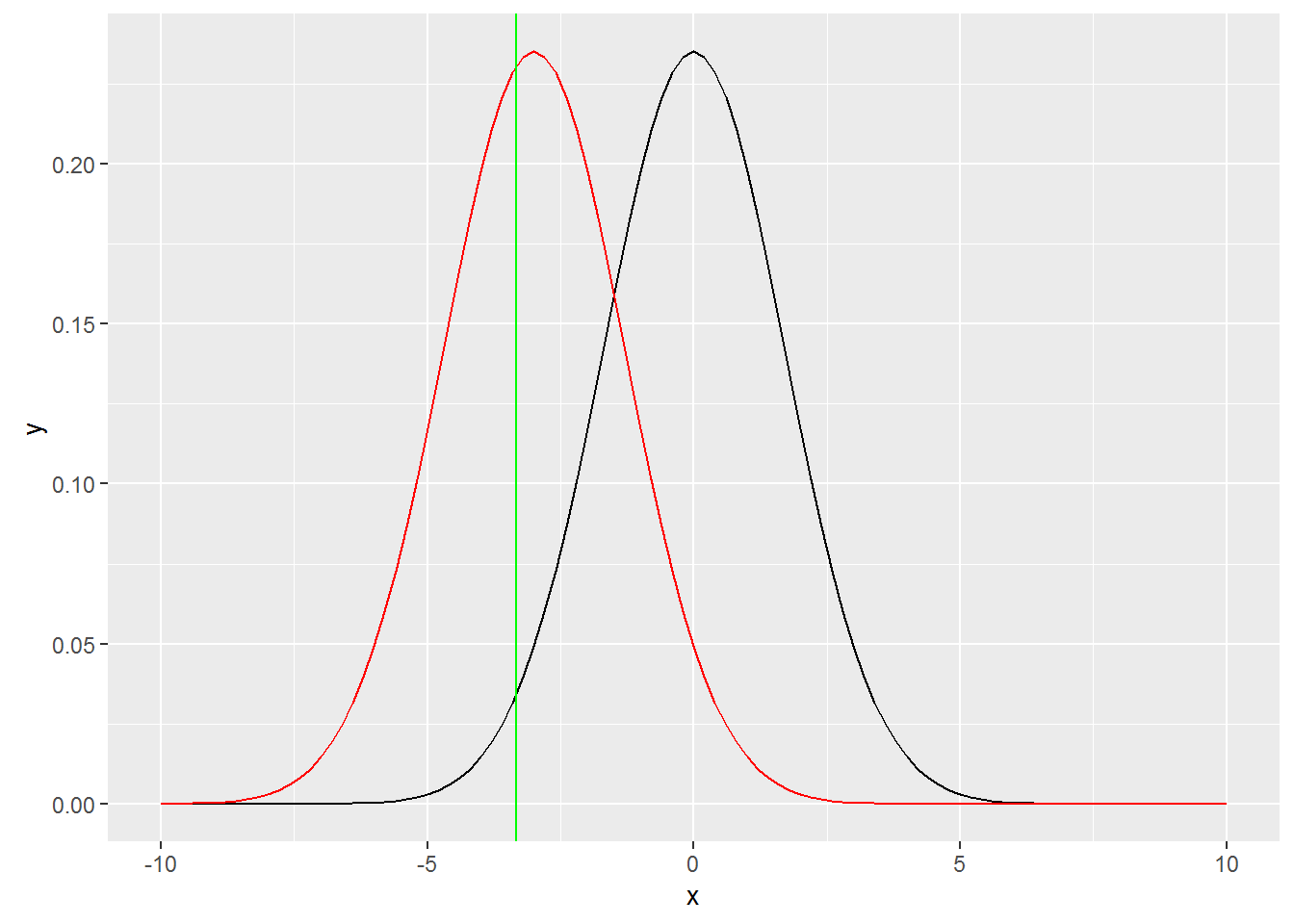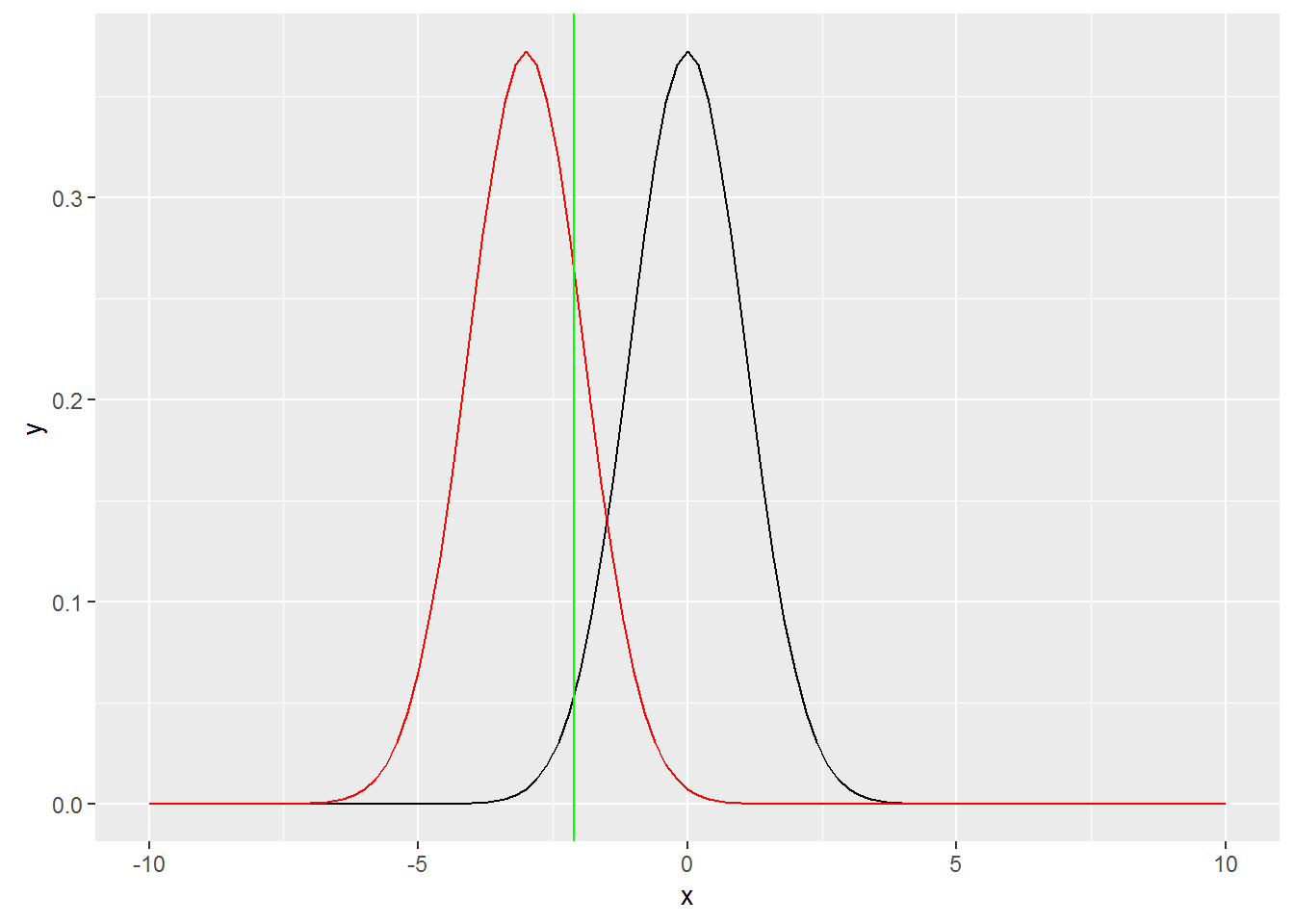# Power and sample size

If you see an error in the article, please comment or drop me an email.

You can calculate the required sample size for a targeted level of power…

…or the obtained power with a given sample size.

# Calculate the obtained power with a given sample size

## 1) Hypotheses

H_0 : mu_diff = 0

H_A : mu_diff != 0

This makes it a two-sided test.

## 2) Draw the rejection zone

``````# In this example, we have a symmetric distribution with the following attributes:
sd <- 12
n <- 100

# We set the significance level at 5%
alpha <- .05
sides <- 2 # it's a two-sided test

# Let us calculate the standard error for two independent means with equal variance...
SE <- sqrt((sd^2/n)+(sd^2/n))

# ...and now the z-score of the right end of the rejection zone
z_score <- qnorm(p=1-(alpha/sides),lower.tail=TRUE) #

# This only to show that the rejection zone
rejectionzone <- c(-1,1) * z_score * SE``````

## 3) Draw the zone around the effect of interest

``````# Our effect_of_interest is negative...
effect_of_interest <- -3

# ...and is therefore left of the rejection zone
left_end_rejectionzone <- (-1) * z_score * SE
diff <- (left_end_rejectionzone - effect_of_interest)/SE

# Power stands for the density of the red distribution centered around the effect of interest and lower than the left end of the rejection zone
power <- pnorm(diff,lower.tail=TRUE)

# As the effect_of_interest is lower than the H_0, the zone around the effect of interest is left of the rejection zone
library(ggplot2)
tmplot <- ggplot(data.frame(x = c(-10, 10)), aes(x))
tmplot <- tmplot + stat_function(fun = dnorm,args = list(sd=SE))
tmplot <- tmplot + stat_function(fun = dnorm,args = list(mean = effect_of_interest,sd=SE),colour="red")
tmplot <- tmplot + geom_vline(xintercept=left_end_rejectionzone,colour="green")
tmplot``````At 42.38%, power is not enough. Let us assume we aim for a power level of 80%.

# Calculate the obtained power with a given sample size

## Redefine the standard error

The effect of interest and the center of the rejection zone do not change.

However, if we want to set the power level at 80%, the left end of the rejection zone should be at the 80th percentile of the distribution centered around the effect of interest.

To make this happen, we need to change the standard error. We can do so by modifying the sample size.

First, let us calculate the distance between the 80th percentile and 0, expressed in standard errors.

The distance between the effect of interest and the left end of the rejection zone is .84 SE

``qnorm(p=.80,lower.tail = TRUE)``
``##  0.8416212``

the distance between the left end of the rejection zone and zero is 1.96 SE

``qnorm(p=.975,lower.tail = TRUE)``
``##  1.959964``

Both distances combined (2.8 SE) are equal to the distance between the effect of interest and zero, hence 3.

``abs(effect_of_interest - 0)``
``##  3``

We can solve for SE

``````# 1.96 * SE + 0.84 * SE = 2.8 * SE = 3
SE <- 3/2.8``````

We can solve for SE

``````# SE = sqrt((sd^2/n)+(sd^2/n))
# SE^2 = (sd^2/n)+(sd^2/n)
# SE^2 = (2 *  sd^2) / n
# n = (2 *  sd^2) /SE^2
n <- (2 *  sd^2) /SE^2
print(n)``````
``##  250.88``

We therefore need 251 samples to obtain a power level of 80% for an effect of interest of 3.

Now let us plot the situation again, with the modified standard error.

``````left_end_rejectionzone <- (-1) * z_score * SE
tmplot <- ggplot(data.frame(x = c(-10, 10)), aes(x))
tmplot <- tmplot + stat_function(fun = dnorm,args = list(sd=SE))
tmplot <- tmplot + stat_function(fun = dnorm,args = list(mean = effect_of_interest,sd=SE),colour="red")
tmplot <- tmplot + geom_vline(xintercept=left_end_rejectionzone,colour="green")
tmplot``````# REVIEW THIS

Power = 1 – beta = Prob( X’ > mu_0 + z_(1-alpha) * sigma/sqrt(n)) sqrt(n)*(mu_a – mu_0) /sigma (mu_a – mu_0)/sigma = EFFECT SIZE power.t.test(n = 16, delta = 2 / 4, sd=1, type = “one.sample”, alt = “one.sided”)\$power Keeping the effect size (ratio delta/sd) constant preserves power

power.t.test(power = .8, delta = 2 / 4, sd=1, type = “one.sample”, alt = “one.sided”)\$n